# C# Richtextbox change font color, add and foreach line, select text, line, display the first n lines

Lionsure 2020-02-24 Original by the website

Richtextbox control is a rich text edit box in C#, which is used to edit rich text. Of course, you can also use it to display text, as long as its background color is set to match the background of the control in which it is. There are two ways to create Richtextbox control, one is to create with controls in toolbar, and the other is to create with code. It has many properties, and setting or modifying them can be used in both the Properties dialog box and the code. This article mainly discusses the latter.

Set or modify the font and color of text in richtextbox control, you can use both specific color names and RGB. The number of lines displayed in Richtextbox and the number of lines separated by a newline character are not a concept. Its attribute lines refers to the latter, while adding, deleting and read lines and displaying a specified number of words per line refer to the former. If you want to highlight the selected text, you can use the highlighted color, large font, or both; in addition to marking one or more lines, you can also mark a specific phrase.

### I, C# Winform create a Richtextbox control

1. Create it from the Toolbox. Drag a Richtextbox control from the Toolbox to the Form to create a Richtextbox control.

2. Create it with code.

Create a Richtextbox control, define several properties at the same time, and add it to the existing Form. The code is as follows:

using System.Drawing;
using System.Windows.Forms;

private void CreateRichTextBoxControl()
{
RichTextBox richTextBox1 = new RichTextBox
{
Dock = DockStyle.None,// No filling, to fill the entire Form with Dock = DockStyle.Fill
Font = new Font("Cambria", 10),
ForeColor = Color.Gray,
Size = new Size(640, 480)
};
richTextBox1.Text = "Create a Richtextbox control";
richTextBox1.Location = new Point(12, 12);
}

If you want to add the created Richtextbox control to a new Form, just change the this.Controls.Add(richTextBox1); to the following code:

Form form = new Form { Controls = { richTextBox1 } };
form.Width = 800;
form.Height = 600;
form.ShowDialog();

Called in the constructed function in Form:

public richtext()
{
InitializeComponent();
CreateRichTextBoxControl();
}

### II, Set style of C# Richtextbox text

1. Richtextbox font color

You can define the font color when creating a Richtextbox, such as ForeColor = Color.Gray above; you can also define it after creating a Richtextbox, the code is as follows:

richTextBox1.ForeColor = Color.DimGray;

or

richTextBox1.ForeColor = Color.FromArgb(255, 108, 105, 105);

FromArgb's first parameter is alpha(transparency), and the last three parameters are R, G, and B (red, green, and blue).

2. Richtextbox font size

Font font = new Font("Cambria", 10F, FontStyle.Regular, GraphicsUnit.Point);
richTextBox1.Font = font;

C# richtextbox bold. If you want to set text to bold, just change the FontStyle.Regular to FontStyle.Bold, that is:

Font font = new Font("Cambria", 10F, FontStyle.Bold, GraphicsUnit.Point);

3. Richtextbox scale factor ZoomFactor

Modify ZoomFactor, the text and line height will automatically increase, for example, change its value to 1.2, see the following code:

richTextBox1.ZoomFactor = (float)1.2;

The effect is shown in Figure 1: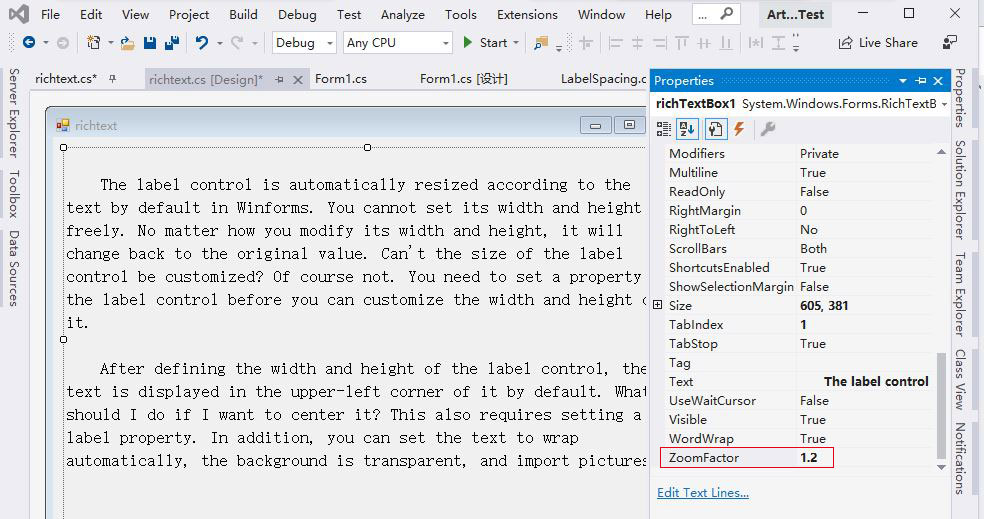Figure 1

### III, C# Richtextbox color line

1. Add new line to the end

richTextBox1.AppendText( Environment.NewLine); or richTextBox1.AppendText("\r\n");

(2) Add a line of text

\r\n is a newline character, placed after the text to indicate a new line from there; if you want to wrap before the text, just put it before the text.

2. Insert a new line

Insert a new line into the first line:

richTextBox1.Text = richTextBox1.Text.Insert(0, "Insert a new line into the first line:\r\n");

0 means insert into the first line. If you want to insert into other lines, change 0 to the corresponding line number. For example, insert into line 6 and change 0 to 6.

3. If the content of RichTextBox is empty, add it to the first line, otherwise add it to the end, the code is as follows:

{
if (String.IsNullOrEmpty(richTextBox1.Text))
richTextBox1.AppendText(text);
else
richTextBox1.AppendText( Environment.NewLine + text);

richTextBox1.ScrollToCaret();// Scroll the content of the control to the current caret position
}

(II) C# Winform Richtextbox adds new lines and text is colored

Suppose you want to display a line of text in three colors. Adding text still uses the AppendText method, but the default method does not have this feature and requires overloading the extension. The code is as follows:

using System;
using System.Windows.Forms;
using System.Drawing;

namespace PublicClass
{
public static class RichTextBoxColorExtensions
{
public static void AppendText(this RichTextBox rtb, string text, Color color, Font font, bool isNewLine = false)
{
rtb.SuspendLayout();
rtb.SelectionStart = rtb.TextLength;
rtb.SelectionLength = 0;

rtb.SelectionColor = color;
rtb.SelectionFont = font;
rtb.AppendText(isNewLine ? \$"{text}{ Environment.NewLine}" : text);
rtb.SelectionColor = rtb.ForeColor;
rtb.ScrollToCaret();
rtb.ResumeLayout();
}
}
}

// Add a new line and the text is colored
private void DifferentColorForRichTextBox()
{
string text = "Orange|1\$/lb|2050Kg||Apple|1.3\$/lb|685Kg||Grape|3.99\$/lb|1258Kg";
string[] arr = text.Split(new string[] { "||" }, StringSplitOptions.RemoveEmptyEntries);
Font font = new Font("Cambria", 12);
string[] subArr;

for (int i = 0; i < arr.Length; i++)
{
subArr = arr[i].Split('|');
richTextBox1.AppendText(subArr, Color.Fuchsia, font);
richTextBox1.AppendText(":");
richTextBox1.AppendText(subArr, Color.Green, font);
richTextBox1.AppendText(" ");
richTextBox1.AppendText(subArr, Color.Blue, font, true);
}
}

Called in the constructed function in Form:

public richtext()
{
InitializeComponent();
DifferentColorForRichTextBox();
}

The effect is shown in Figure 2: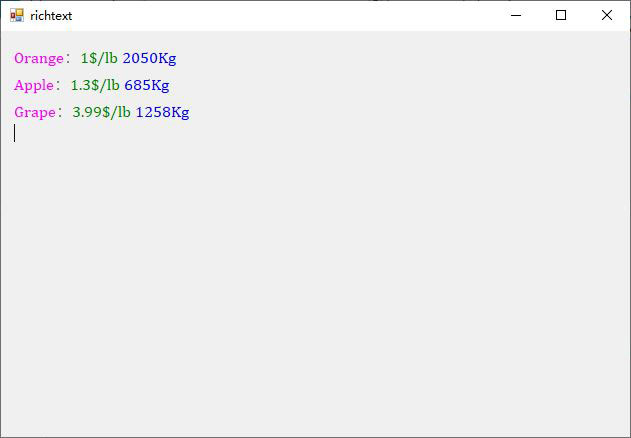Figure 2

(III) C# Winform Richtextbox scroll to the last line

richTextBox1.SelectionStart = richTextBox1.TextLength;// or richTextBox1.Select(richTextBox1.TextLength, 0);
richTextBox1.ScrollToCaret();
richTextBox1.AppendText(richTextBox1.Text);

(IV) C# Richtextbox lines

1. RichTextBox.Lines mean

RichTextBox.Lines refers to the number of text lines to the right of Text in the Properties dialog box, not the number of text lines in the Form. If there are two lines of text in RichTextBox1, as shown in Figure 3: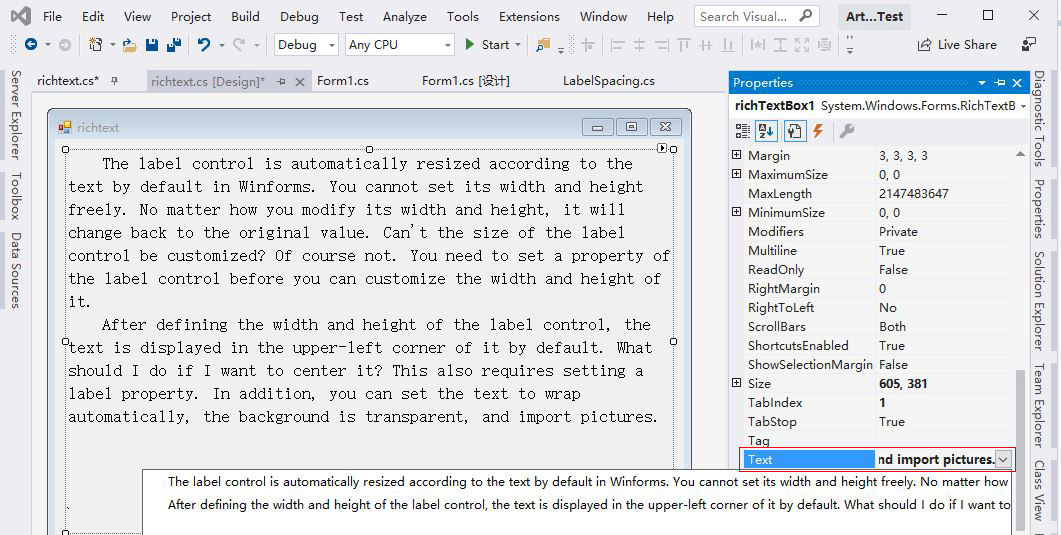Figure 3

Use the code:

int n = richTextBox1.Lines.Length;

The number of rows is displayed, and the result is 2, as shown in Figure 4: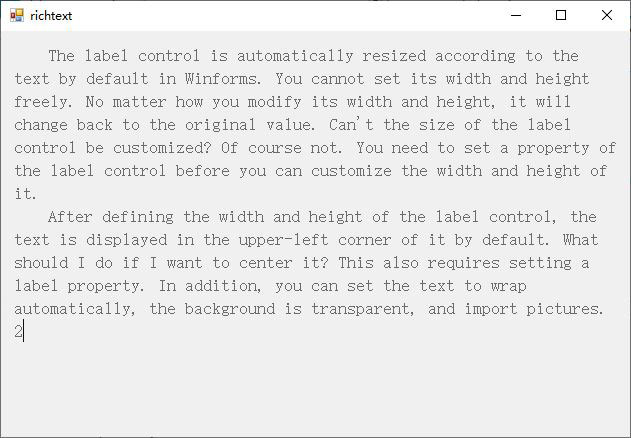Figure 4

2. Read RichTextBox.Lines of text line by line(Foreach line in richtextbox C#)

{
foreach(string l in richTextBox1.Lines)
{
MessageBox.Show(l);
}
}

If you want to read the line containing Winforms, the code is as follows:

{
// Find where the specified text is
int pos = richTextBox1.Find(specialText, RichTextBoxFinds.MatchCase);
if (pos != -1)
{
// Returns the line number based on the index of specified character
int numLine = richTextBox1.GetLineFromCharIndex(pos);
string text = richTextBox1.Lines.ElementAt(numLine);
return text;
}
return null;
}

Call:

You can also use Lambda expression, the code is as follows:

{
return richTextBox1.Lines.FirstOrDefault(l => l.Contains(specialText));
}

4. RichTextBox displays only the first n lines

(1) Display the first n lines, you cannot specify the number of characters in each line

private void RichTextBoxShowNLines(int numLine)
{
richTextBox1.Lines = richTextBox1.Lines.Take(numLine).ToArray();
}

Call (show only the first 5 lines):

RichTextBoxShowNLines(5);

(2) Display the first n lines, can specify the number of characters per line

/// <summary>
/// Show only the first n lines and specify the number of characters per line
/// </summary>
/// <param name="text">text</param>
/// <param name="numLine">Display the number of rows</param>
/// <param name="numPerLine">Characters per line</param>
/// <param name="replaceLineBreak">Whether to replace newlines in text</param>
/// <returns>Text with specified number of lines per line</returns>

private string ShowNLinesInRichTextBox(string text, int numLine, int numPerLine, bool replaceLineBreak = false)
{
if (string.IsNullOrEmpty(text) || numLine <= 0 || numPerLine <= 0)
return null;
if (text.Length <= numPerLine)
return text;// When the number of text is less than the number of characters required per line, the original text is returned

string newLine = Environment.NewLine;
if (replaceLineBreak)
{
text = text.Replace("\n", "").Replace("\r", "");
int n = text.Length / numPerLine;
if (text.Length % numPerLine > 0)
n++;

if (n > numLine)
n = numLine;

// Insert newlines after the specified number of characters per line
for (int i = 0; i < n; i++)
{
if (i == 0)
{
if (i == n - 1)// Show only one line
text = text.Substring(0, numPerLine);
else
text = text.Insert(numPerLine, newLine);
}
else
{
if (i != n - 1)// No line breaks n the last line
text = text.Insert((i + 1) * numPerLine + i * newLine.Length, newLine);
else
{
// If the original text is longer than the specified number of lines, only the specified number of lines are displayed, (i - 1)* newLine.Length is the number of newline characters inserted
if (text.Length >= (i + 1) * numPerLine + i * newLine.Length)
text = text.Substring(0, (i + 1) * numPerLine + i * newLine.Length);
}
}
}
}
else
{
string[] arr = text.Split(Environment.NewLine.ToCharArray(), StringSplitOptions.RemoveEmptyEntries);
int currLineNum = 0;
text = string.Empty;
for (int i = 0; i < arr.Length && currLineNum < numLine; i++)
{
if (!string.IsNullOrEmpty(arr[i]))
{
int n = arr[i].Length / numPerLine;
if (arr[i].Length % numPerLine > 0)
n++;
if (n == 0)
n = 1;

bool flag = false;// Whether a newline was inserted last time
for (int j = 0; j < n && currLineNum < numLine; j++)
{
// Re-split into multiple lines only if the number of characters per line of the original text is greater than the specified number of characters
if (arr[i].Length > numPerLine)
{
if (j == 0)
{
if (currLineNum == numLine - 1)
arr[i] = arr[i].Substring(0, numPerLine);// Show only one line
else
arr[i] = arr[i].Insert(numPerLine, newLine);
}
else
{
// Insert a newline after each line of the specified number of characters
if (currLineNum != n - 1 && j != numLine - 1)
arr[i] = arr[i].Insert((j + 1) * numPerLine + j * newLine.Length, newLine);
else
{
if ((j + 1) * numPerLine + j * newLine.Length < arr[i].Length)
{
arr[i] = arr[i].Insert((j + 1) * numPerLine + j * newLine.Length, newLine);
flag = true;
}
}
// Intercept from the current line of the original text to the last line
if (currLineNum == numLine - 1 && flag)
arr[i] = arr[i].Substring(0, arr[i].LastIndexOf(newLine));
}
}
currLineNum++;
}
}
text += arr[i];
if (currLineNum != numLine - 1)// Not the last line, add a newline after each paragraph
text += newLine;
}
}
return text;
}

Call:

// Replace line breaks in the original text:
richTextBox1.Text = ShowNLinesInRichTextBox(richTextBox1.Text, 9, 62, true);

// Do not replace line breaks in the original text:
richTextBox1.Text = ShowNLinesInRichTextBox(richTextBox1.Text, 9, 62);

The effect is shown in Figure 5: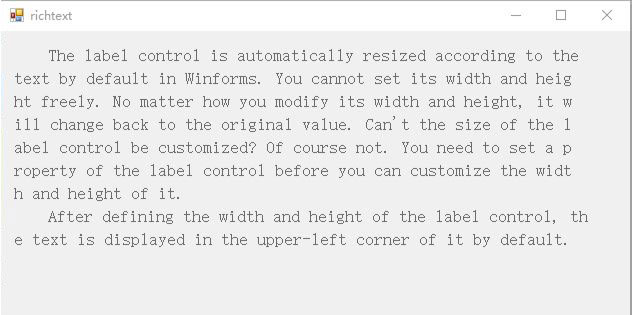Figure 5

(V) C# Richtextbox clear text

1. Clear RichTextBox

richTextBox1.Clear();

2. Delete any specified line

/// <summary>
/// Delete any specified line
/// </summary>
/// <param name="startLineNum">Start line number</param>
/// <param name="endLineNum">End line number</param>

private void DeleteSpecialLines(int startLineNum, int endLineNum)
{
// Get the index of the first character in the first line in the specified line
int start = richTextBox1.GetFirstCharIndexFromLine(startLineNum - 1);

// Get the index of the first character in the last line in the specified lines
int end = richTextBox1.GetFirstCharIndexFromLine(endLineNum);
richTextBox1.Select(start, end - start);// Select the specified line
richTextBox1.SelectedText = "";// Set the content of the selected row to empty
}

Call:

DeleteSpecialLines(1, 1);// Delete line 1

DeleteSpecialLines(6, 6);// Delete line 6

DeleteSpecialLines(2, 4);// Delete lines 2 to 4

3. Delete the last line

richTextBox1.Text = richTextBox1.Text.Remove(richTextBox1.Text.Length - 1);

### IV, C# Richtextbox change font selected text

1. Right-click Richtextbox, select "Properties" from the pop-up menu, open the "Properties" dialog box, select the "Events" tab, add richTextBox1_SelectionChanged to the right of SelectionChanged, and then press Enter, adds a selection event for it, as shown in Figure 6: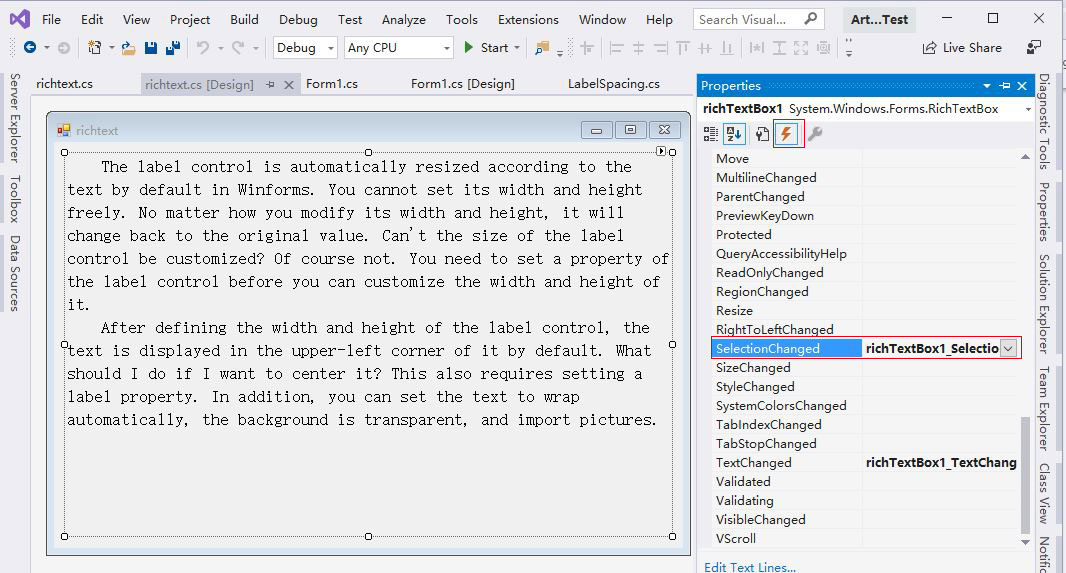Figure 6

2. Then add the following code:

private void richTextBox1_SelectionChanged(object sender, EventArgs e)
{
if (richTextBox1.SelectedText.Length > 0)
{
richTextBox1.SelectionFont = new Font("Cambria", 14);
richTextBox1.SelectionColor = Color.Red;
}
}

### V, C# Richtextbox change text color

1. Change the line containing the specified text to the specified color

If you want to change the line containing Label to the specified color(such as "red"), the code is as follows:

private void ChangeColorForSpecialText(string specialText, Color color)
{
if (string.IsNullOrEmpty(specialText))
return;

int pos = 0;
int numLine;
List<int> lst = new List<int>();
do
{
if (pos != 0 && pos + specialText.Length < richTextBox1.Text.Length)

// Find the index of specified text
pos = richTextBox1.Find(specialText, pos, RichTextBoxFinds.None);

if (pos > 0)
{
// Returns the line number of text based on its index
numLine = richTextBox1.GetLineFromCharIndex(pos);
}
if (pos + specialText.Length > richTextBox1.Text.Length - 1)
break;
}while (pos >= 0 && pos < richTextBox1.Text.Length);

for (int i = 0; i < lst.Count; i++)
{
SelectLine(lst[i]);
richTextBox1.SelectionColor = color;
}
}

// Select a line, numLine is the line number
private void SelectLine(int numLine)
{
if (numLine < 0)
return;

// Returns the index of the first character in the line based on the line number
int start = this.richTextBox1.GetFirstCharIndexFromLine(numLine);
int end = this.richTextBox1.GetFirstCharIndexFromLine(++numLine);
if (start == -1)
return;
else if (end == -1)// If end exceeds length of text, use length of text - start
end = this.richTextBox1.TextLength - start;
else
end = end - start;
this.richTextBox1.Select(start, end);
}

Call:

ChangeColorForSpecialText("Label", Color.Red);

The effect is shown in Figure 7: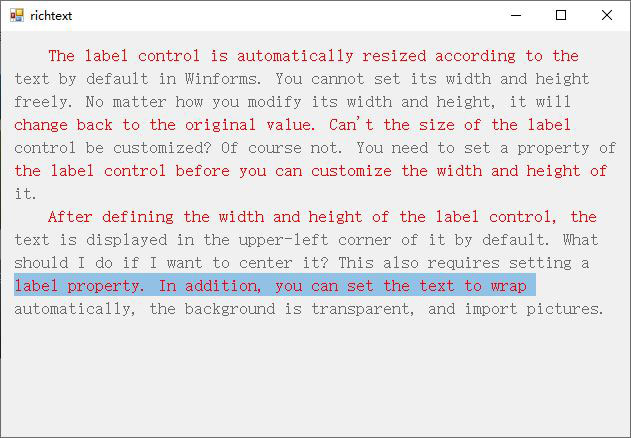Figure 7

2. Change the specified text to the specified color

private void ChangeColorForSpecialWord(string specialWord, Color color)
{
if (string.IsNullOrEmpty(specialWord))
return;

int pos = 0;
do
{
if (pos != 0 && pos + specialWord.Length < richTextBox1.Text.Length)

// Find the index of a specified word
pos = richTextBox1.Find(specialWord, pos, RichTextBoxFinds.None);
if (pos > 0)
{
richTextBox1.Select(pos, specialWord.Length);
richTextBox1.SelectionColor = color;
}
if (pos + specialWord.Length > richTextBox1.Text.Length - 1)
break;
} while (pos >= 0 && pos < richTextBox1.Text.Length);
}

Call:

ChangeColorForSpecialWord("Control", Color.Red);// Control is marked in red

The effect is shown in Figure 8: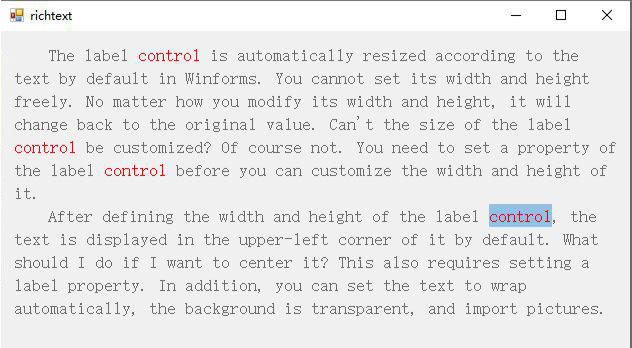Figure 8

// Words across lines are marked in red(search across lines)
ChangeColorForSpecialWord("it.\r After", Color.Red);

The effect is shown in Figure 9: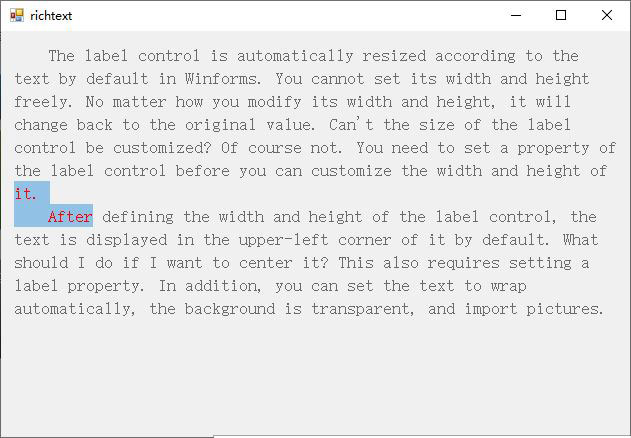Figure 9